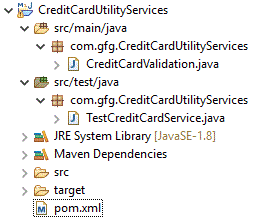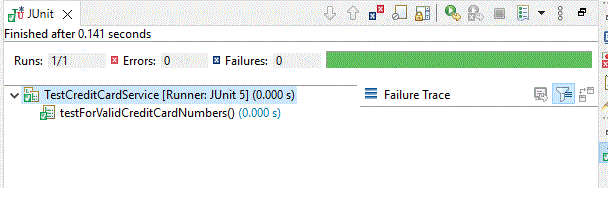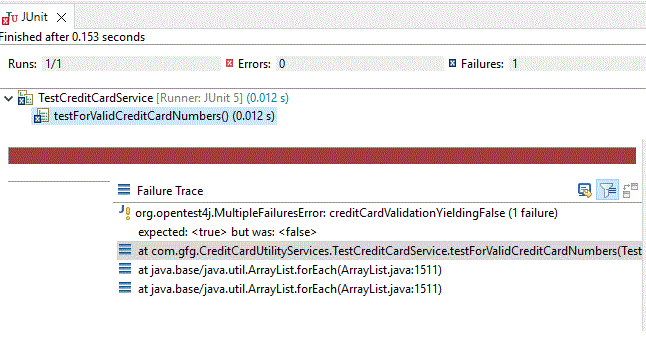Open in App
Not now

# JUnit – Testcases for Credit Card Validation as a Maven Project

• Last Updated : 15 Nov, 2022

In this article let us see a much-required functionality that is credit card validation as well as its relevant JUnit test cases for given credit card numbers. In an e-commerce project, credit card payment is the valid and much desired required functionality. We need to have a proper validation mechanism for that. So, by using the “Luhn algorithm”, the validation code is done. As a maven project, let us cover the validation as well as the JUnit test case part.

### Sample Maven Project

Project Structure:Dependencies need to be specified in

pom.xml

## XML

 ` ` `<``project` `xmlns``=``"http://maven.apache.org/POM/4.0.0"` `         ``xmlns:xsi``=``"http://www.w3.org/2001/XMLSchema-instance"` `         ``xsi:schemaLocation="http://maven.apache.org/POM/4.0.0 ` `                             ``http://maven.apache.org/xsd/maven-4.0.0.xsd"> ` ` `  `    ``<``modelVersion``>4.0.0 ` `    ``<``groupId``>com.gfg.CreditCardUtilityServices ` `    ``<``artifactId``>CreditCardUtilityServices ` `    ``<``packaging``>jar ` `    ``<``version``>1.0-SNAPSHOT ` ` `  `    ``<``properties``> ` `        ``` `        ``<``project.build.sourceEncoding``>UTF-8 ` `        ``<``maven.compiler.source``>1.8 ` `        ``<``maven.compiler.target``>1.8 ` `        ``<``junit.version``>5.3.1 ` `        ``<``pitest.version``>1.4.3 ` `    `` ` ` `  `    ``<``dependencies``> ` ` `  `        ``` `        ``<``dependency``> ` `            ``<``groupId``>org.junit.jupiter ` `            ``<``artifactId``>junit-jupiter-engine ` `            ``<``version``>\${junit.version} ` `            ``<``scope``>test ` `        `` ` ` `  `    `` ` `    ``<``build``> ` `        ``<``finalName``>UtilityServices ` `        ``<``plugins``> ` `            ``<``plugin``> ` `                ``<``groupId``>org.apache.maven.plugins ` `                ``<``artifactId``>maven-surefire-plugin ` `                ``<``version``>3.0.0-M1 ` `            `` ` ` `  `            ``<``plugin``> ` `                ``<``groupId``>org.pitest ` `                ``<``artifactId``>pitest-maven ` `                ``<``version``>\${pitest.version} ` ` `  `                ``<``executions``> ` `                    ``<``execution``> ` `                        ``<``id``>pit-report ` `                        ``<``phase``>test ` `                        ``<``goals``> ` `                            ``<``goal``>mutationCoverage ` `                        `` ` `                    `` ` `                `` ` ` `  `                ``` `                ``` `                ``<``dependencies``> ` `                    ``<``dependency``> ` `                        ``<``groupId``>org.pitest ` `                        ``<``artifactId``>pitest-junit5-plugin ` `                        ``<``version``>0.8 ` `                    `` ` `                `` ` `                ``<``configuration``> ` `                    ``<``targetClasses``> ` `                        ``<``param``>com.gfg.CreditCardUtilityServices.*CreditCardUtilityServices* ` `                         `  `                    `` ` `                    ``<``targetTests``> ` `                        ``<``param``>com.gfg.CreditCardUtilityServices.* ` `                    `` ` `                `` ` `            `` ` ` `  `        `` ` `    `` ` ` `  ` `

Now let us write the business logic of validating a credit card

CreditCardValidation.java

## Java

 `public` `class` `CreditCardValidation {    ` `  `  `    ``/* Return true if the card number is valid by using Luhn algorithm. ` `    ``Prerequisites ` `        ``A credit card number must have between 13 and 16 digits.  ` `        ``It must start with: ` `        ``4 for Visa cards ` `        ``5 for Master cards ` `        ``37 for American Express cards ` `        ``6 for Discover cards ` `    ``*/` `    ``public` `static` `boolean` `checkForValidity(``long` `number) ` `    ``{ ` `       ``return` `(getNumberOfDigits(number) >= ``13` `&& ` `               ``getNumberOfDigits(number) <= ``16``) && ` `               ``(checkForPrefixMatching(number, ``4``) || ` `                ``checkForPrefixMatching(number, ``5``) || ` `                ``checkForPrefixMatching(number, ``37``) || ` `                ``checkForPrefixMatching(number, ``6``)) && ` `              ``((sumOfDoubleEvenPlaces(number) + ` `                ``getSumOfOddPlaces(number)) % ``10` `== ``0``); ` `    ``} ` `  `  `    ``// Get the result from Step 2 ` `    ``public` `static` `int` `sumOfDoubleEvenPlaces(``long` `inputNumber) ` `    ``{ ` `        ``int` `summation = ``0``; ` `        ``String number = inputNumber + ``""``; ` `        ``for` `(``int` `i = getNumberOfDigits(inputNumber) - ``2``; i >= ``0``; i -= ``2``) ` `            ``summation += getTheDigits(Integer.parseInt(number.charAt(i) + ``""``) * ``2``); ` `          `  `        ``return` `summation; ` `    ``} ` `  `  `    ``// When the input number is a single digit that  ` `    ``// means between 0 to 9, Return this number as it is ` `    ``// If not,  ` `    ``// return the sum of the two digits ` `    ``public` `static` `int` `getTheDigits(``int` `inputNumber) ` `    ``{ ` `        ``if` `(inputNumber < ``9``) ` `            ``return` `inputNumber; ` `        ``return` `inputNumber / ``10` `+ inputNumber % ``10``; ` `    ``} ` `  `  `    ``// We are going to add the odd-place digits  ` `      ``// in number and return their sum ` `    ``public` `static` `int` `getSumOfOddPlaces(``long` `inputnumber) ` `    ``{ ` `        ``int` `summation = ``0``; ` `        ``String number = inputnumber + ``""``; ` `        ``// As odd places, we need to decrement by 2 ` `        ``for` `(``int` `i = getNumberOfDigits(inputnumber) - ``1``; i >= ``0``; i -= ``2``) ` `            ``summation += Integer.parseInt(number.charAt(i) + ``""``);        ` `        ``return` `summation; ` `    ``} ` `  `  `    ``// Return true if the digit  is a prefix for number ` `    ``public` `static` `boolean` `checkForPrefixMatching(``long` `inputnumber, ``int` `digit) ` `    ``{ ` `        ``return` `getPrefixNumber(inputnumber, getNumberOfDigits(digit)) == digit; ` `    ``} ` `  `  `    ``// Return the number of digits ` `    ``public` `static` `int` `getNumberOfDigits(``long` `digit) ` `    ``{ ` `        ``String number = digit + ``""``; ` `        ``return` `number.length(); ` `    ``} ` `  `  `    ``// By using substring functionality, we can  ` `    ``// return the first k number of digits from ` `    ``// number. If the number of digits in number ` `    ``// is less than k, return number. ` `    ``public` `static` `long` `getPrefixNumber(``long` `inputnumber, ``int` `k) ` `    ``{ ` `        ``if` `(getNumberOfDigits(inputnumber) > k) { ` `            ``String num = inputnumber + ``""``; ` `            ``return` `Long.parseLong(num.substring(``0``, k)); ` `        ``} ` `        ``return` `inputnumber; ` `    ``} ` `} `

Now let us go with JUNIT test cases. It covers a few valid test credit card numbers from “American Express, Diners Club, Master, Visa card, etc.,”

TestCreditCardService.java

## Java

 `import` `static` `org.junit.jupiter.api.Assertions.assertAll; ` `import` `static` `org.junit.jupiter.api.Assertions.assertArrayEquals; ` `import` `static` `org.junit.jupiter.api.Assertions.assertEquals; ` `import` `static` `org.junit.jupiter.api.Assertions.assertFalse; ` `import` `static` `org.junit.jupiter.api.Assertions.assertNotEquals; ` `import` `static` `org.junit.jupiter.api.Assertions.assertTrue; ` `import` `static` `org.junit.jupiter.api.Assumptions.assumeFalse; ` `import` `static` `org.junit.jupiter.api.Assumptions.assumingThat; ` ` `  `import` `org.junit.jupiter.api.Assertions; ` `import` `org.junit.jupiter.api.Test; ` ` `  `public` `class` `TestCreditCardService { ` `    ``@Test` `    ``public` `void` `testForValidCreditCardNumbers() { ` `        ``// Example for American Express card ` `        ``assertEquals(``true``, CreditCardValidation.checkForValidity(378282246310005L)); ` `        ``assertNotEquals(``true``, CreditCardValidation.checkForValidity(37828224631015L)); ` `           `  `        ``// Example for American Express Corporate card ` `        ``assertEquals(``true``, CreditCardValidation.checkForValidity(378734493671000L)); ` `           `  `        ``// Example for Discover card ` `        ``assertTrue(CreditCardValidation.checkForValidity(6011111111111117L)); ` `        ``assertFalse(CreditCardValidation.checkForValidity(6011111111111128L),``"Invalid Creditcard"``); ` `        ``String validationName = ``"creditCardValidation"``; ` `         `  `         ``// In a grouped assertion all assertions are executed, and any ` `        ``// failures will be reported together. ` `        ``// Example for Master card and Visa ` `        ``assertAll(``"creditCardValidation"``, () -> assertTrue(CreditCardValidation.checkForValidity(5105105105105100L)), ` `                ``() -> assertTrue(CreditCardValidation.checkForValidity(4111111111111111L))); ` `        ``// Let us comment for the first time ` `        ``// assertAll("creditCardValidationYieldingFalse", () -> assertTrue(CreditCardValidation.checkForValidity(378282246310005L)), ` `        ``//                 () -> assertTrue(CreditCardValidation.checkForValidity(378282246310005L))); ` `         `  `        ``/* The assumingThat() method executes the rest of the statements  ` `           ``if the assumption is valid. If the assumption is invalid,  ` `           ``this method does nothing.  ` `           ``Advantage : To log the information ` `        ``*/` `       `  `       ``// Example for Visa card ` `       ``assumingThat(``"creditCardValidation"``.equals(validationName), () -> { ` `            ``System.out.println(``"Checking for creditcard validation!!!"``); ` `            ``assertEquals(``true``, CreditCardValidation.checkForValidity(4012888888881881L)); ` `        ``}); ` `       `  `        ``/* with assumeFalse ` `        ``* If the boolean condition in assumeFalse becomes false  ` `        ``* then only the next set of test method is executed,  ` `        ``* else the test is skipped.  ` `        ``*/`        `        ``assumeFalse(``"loginValidation"``.equals(validationName)); ` `       `  `        ``// Example for Paymentech (Processor specific card) ` `        ``assertTrue(CreditCardValidation.checkForValidity(6331101999990016L)); ` `       `  `        ``// Example for American Express,Diners and Master card (May be 3 people at a home are having 3 different cards,  ` `        ``// we can check in this way, instead of checking single single ` `        ``assertArrayEquals(``new` `long``[]{378282246310005L,30569309025904L,5555555555554444L},``new` `long``[]{378282246310005L,30569309025904L,5555555555554444L}); ` `        ``Assertions.assertThrows(IllegalArgumentException.``class``, () -> { ` `            ``Integer.parseInt(``"378282246310005L"``); ` `        ``}); ` `       `  `    ``}   ` `     `  `}`

Explanation for assertAll:

Sometimes there is a necessity for providing positive results, and multiple conditions to be checked

``` assertAll("creditCardValidation", () -> assertTrue(CreditCardValidation.checkForValidity(5105105105105100L)),
() -> assertTrue(CreditCardValidation.checkForValidity(4111111111111111L)));```

Here two separate assertTrue statements are written and both should be true i.e. given card should be validated as true and then only the whole condition is passed.In the above code, let us uncomment the below code

```assertAll("creditCardValidationYieldingFalse", () -> assertTrue(CreditCardValidation.checkForValidity(378282246310005L)),
() -> assertTrue(CreditCardValidation.checkForValidity(379382246310005L)));```

Among the two cards given, the first one is a valid card and the second is not valid. Hence the whole condition becomes false and we can see that from the result alsoFor doing grouped test results, assertAll is very much helpful.

assumingThat:

There are situations to proceed with the execution of the test case. For that scenario, we can use

```// Example for Visa card
assumingThat("creditCardValidation".equals(validationName), () -> { // Only when the condition is valid
System.out.println("Checking for creditcard validation!!!");// We can log the required information here
assertEquals(true, CreditCardValidation.checkForValidity(4012888888881881L));
});```

Ironically to test something, we can use

assumeFalse:

```/* with assumeFalse
* If the boolean condition in assumeFalse becomes false then only the next set of test method is executed,
* else the test is skipped.
*/

// false here and hence next statement is proceeded
// Example for Paymentech (Processor specific card)
assertTrue(CreditCardValidation.checkForValidity(6331101999990016L));```

assertArrayEquals:

Instead of testing a single card, we can put everything in an array and we can check that.

```// Example for American Express,Diners and Master card (May be 3 people at a home are having 3 different cards, we can check in this way, instead of checking single single
assertArrayEquals(new long[]{378282246310005L,30569309025904L,5555555555554444L},new long[]{378282246310005L,30569309025904L,5555555555554444L});```

Thus JUnit helps a lot to get quality software. By writing efficient JUnits, surely the best software can be produced.

My Personal Notes arrow_drop_up
Related Articles